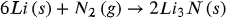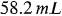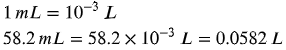1
279
views
46
Problem

# Problem 46

## Chapter 5: GasesTextbook ExpertVerified Tutor
25 Oct 2021

#### Given information

The balanced chemical equation for the given reaction is,The volume of nitrogen gas in the reaction is.

#### Step-by-step explanation

Step 1.

Convert the volume into liter from milliliter and then calculate the number of moles of nitrogen gas by using the ideal gas equation.